• 傅里叶变换是图像处理中常用的技术，用于图像的去噪，该算法是基于matlab语言编写，简单易懂。
• 傅里叶变换matlab实现，初学者学习的好材料。
• 分阶傅里叶变换MATLAB代码，文件中附有详细解释，可供入门学者参考学习。此代码是定义函数，下载后可自行编辑变换对象即可得到变换结果。
• 实现二维傅里叶变换matlab程序，显示原函数和傅里叶变换的三维彩色立体图，
• 时频分析 短时傅里叶变换 Matlab程序 好用 地震信号处理
• 短时傅里叶变换matlab程序 function [Spec,Freq]=STFT(Sig,nLevel,WinLen,SampFreq) %计算离散信号的短时傅里叶变换 % Sig 待分析信号 % nLevel 频率轴长度划分默认值512 % WinLen 汉宁窗长度默认值 64 % SampFreq ...
• 学 海 无 涯 非周期信号的傅里叶变换MATLAB 仿真实验 一实验目的 1熟悉连续非周期信号频谱特点及其分析方法 2 掌握用MATLAB 实现傅里叶变换 二非周期信号的傅里叶变换原理及性质 设周期信号 f (t) 展开成复指数形式...
• 短时傅里叶变换 matlab源程序，供大家参考学习
• 非周期信号的傅里叶变换MATLAB仿真实验 实验目的 熟悉连续非周期信号频谱特点及其分析方法 掌握用MATLAB实现傅里叶变换 非周期信号的傅里叶变换原理及性质 设周期信号展开成复指数形式的傅里叶级数为 两边同乘 得 上...
• 根据一组时程数据，在matlab中通过傅里叶变换求解频率f，并且画出相应的图形，振幅随频率变化的曲线等。
• 本程序是短时傅里叶变换matlab程序，短时傅里叶变换是信号分析的重要手段，在视频，图像，音频信号中有着非常重要的作用。
• 快速傅里叶变换代码，输入时域信号有限离散序列，得到相应的离散傅里叶变换FFT
• 用于傅里叶变换的mabtlab函数 快速傅氏变换，是离散傅氏变换（DFT）的快速算法，它是根据离散傅氏变换的奇、偶、虚、实等特性，对离散傅立叶变换的算法进行改进获得的图像处理
• 读取TXT格式数据文件，进行短时傅里叶变换，并将结果保存为图片的MATLAB脚本。短时傅里叶变换是一种经典的时频分析算法，常用于故障诊断等信号处理与分析领域。
• 包含 4种 分数傅里叶变换matlab代码实现方式，可以用于图像加密，分数傅里叶变换分析
• 快速傅里叶变换程序，后面有滤波程序，每一步有注释
• 短时傅里叶变换（STFT）是和傅里叶变换相关的一种数学变换，用以确定时变信号其局部区域正弦波的频率与相位。
• %傅里叶变换 clc;clear all;close all; tic Fs=128%采样频率频谱图的最大频率 T=1/Fs%采样时间原始信号的时间间隔 L=256%原始信号的长度即原始离散信号的点数 t=(0:L-1*T%原始信号的时间取值范围 x=7*cos(2*pi*15*t-...
• ## 逆傅里叶变换matlab

热门讨论 2010-12-27 19:41:36
给出了一个逆傅里叶变换IFFT的MATLAB函数文件
• 参数可自由选择，可以根据自己需要选择步长、窗函数种类从而实现短时傅里叶变换。 参数可自由选择，可以根据自己需要选择步长、窗函数种类从而实现短时傅里叶变换
• 对现有的音频文件进行傅里叶分析，多个程序，MATLAB编程
• 从数学意义上看，傅里叶变换试讲一个图像转换为一系列周期函数来处理的。从物理效果上看，傅里叶变换从空间域转换到频率域。换句话说傅里叶变换是将图像的灰度分布函数转换为图像的频率分布函数。实际上对图像进行二...
  从数学意义上看，傅里叶变换试讲一个图像转换为一系列周期函数来处理的。从物理效果上看，傅里叶变换从空间域转换到频率域。换句话说傅里叶变换是将图像的灰度分布函数转换为图像的频率分布函数。实际上对图像进行二维傅里叶变换得到频谱图，就是图像梯度的分布图，傅里叶频谱图上看到的明暗不一的亮点就是某一点与领域差异的强弱，即梯度的大小（该点的频率大小）。

一.原理

1、代数形式的傅里叶变换：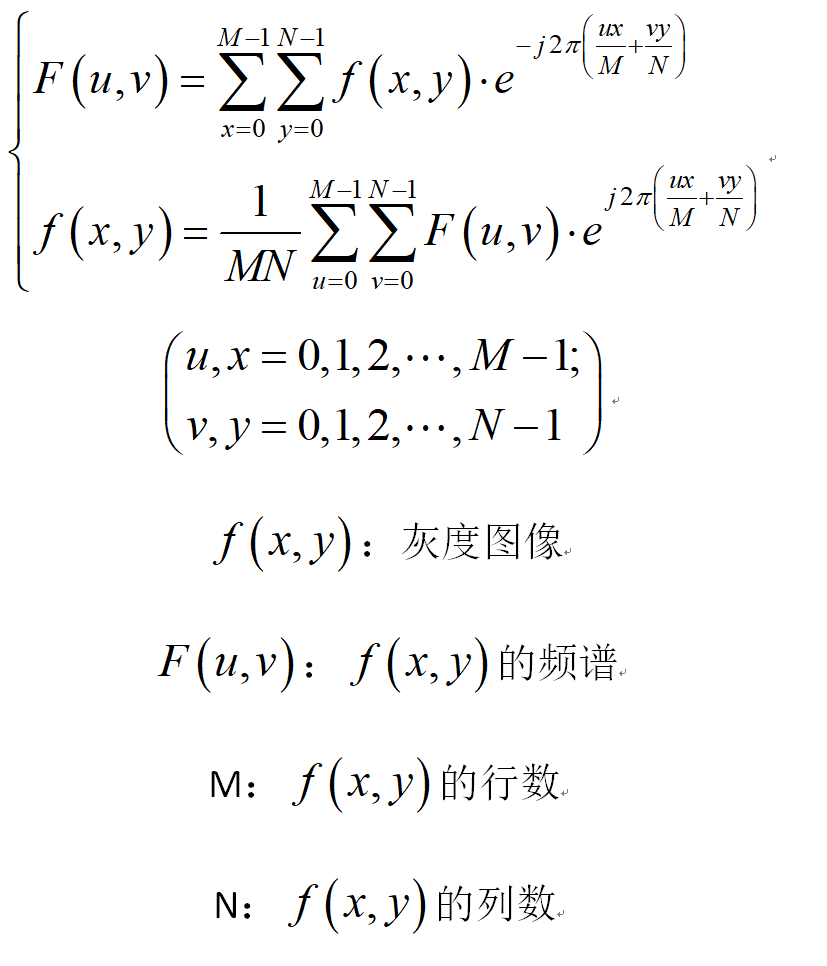2、矩阵形式的二维离散傅里叶变换：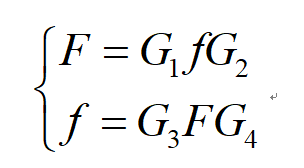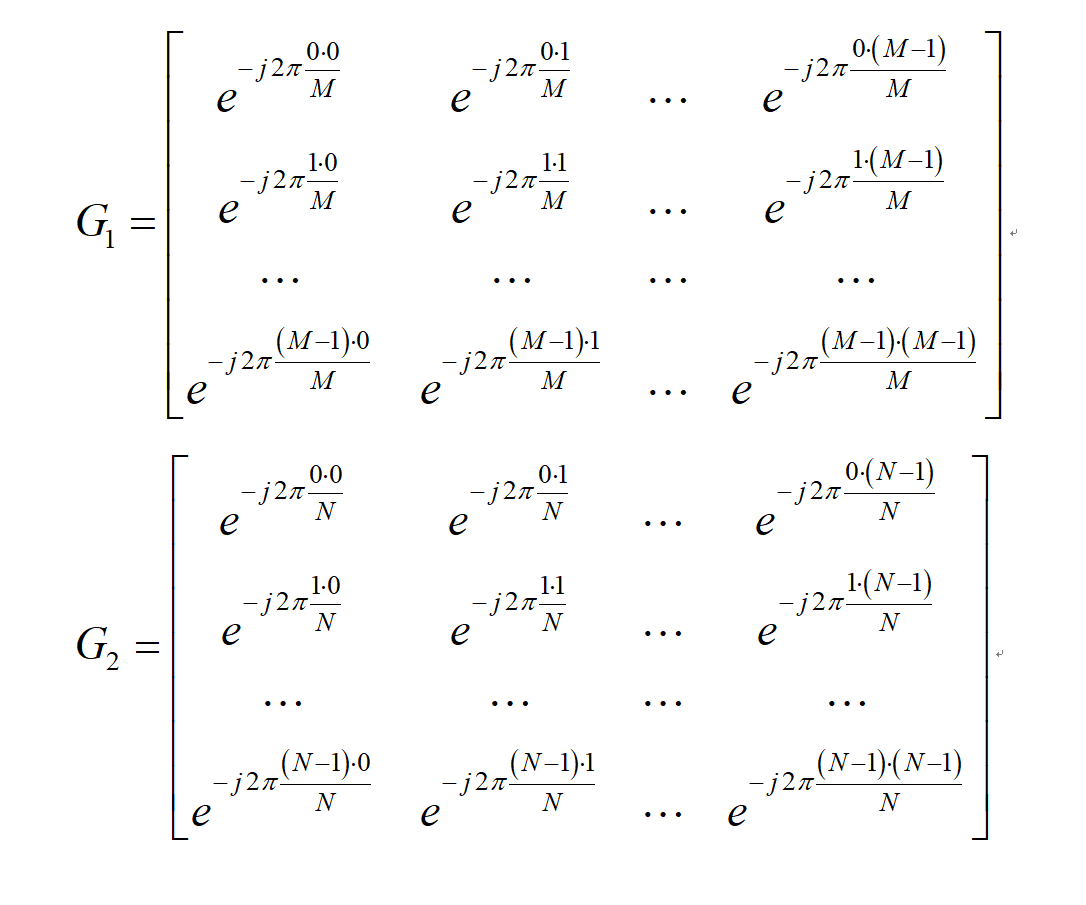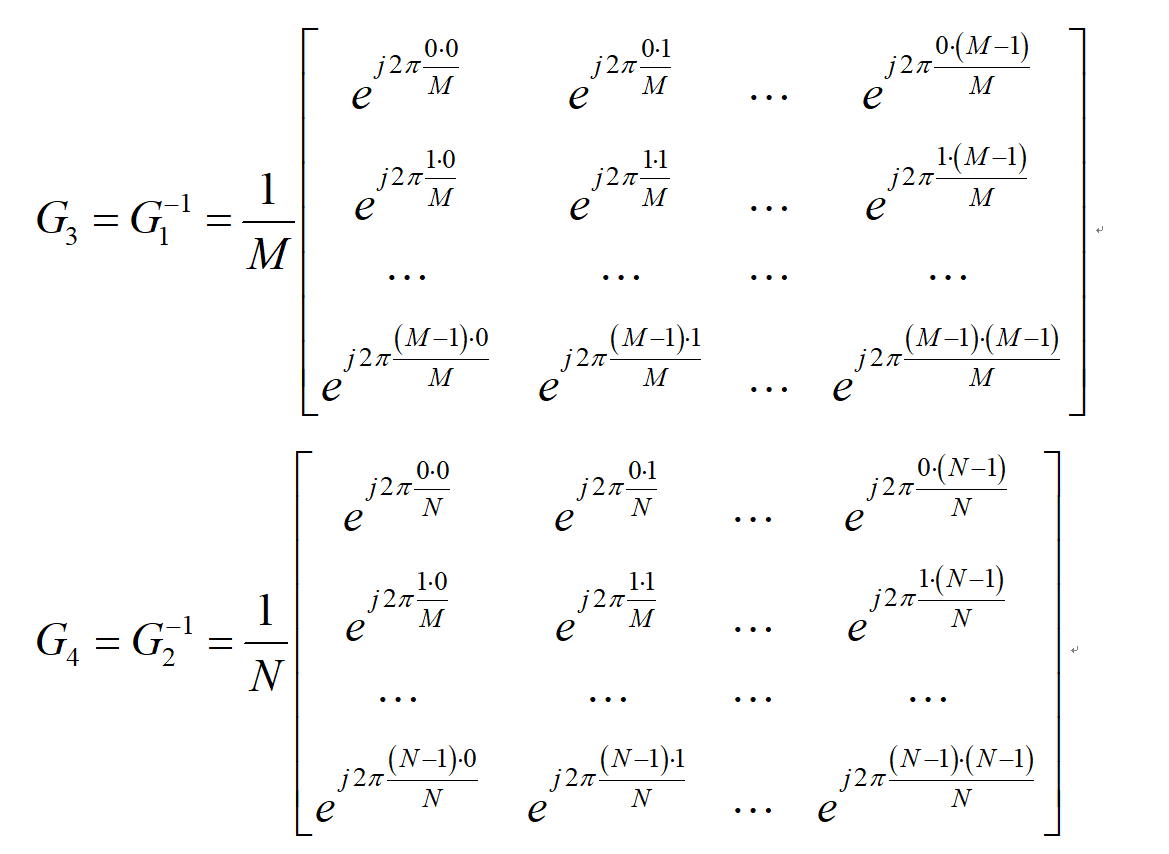傅里叶变换不管是在图像处理中还是在科研中都有着很多的用处。例如在阿贝波特空间滤波实验中，就使用了透镜将图像从空间域转换到频率域进行滤波从而实现一系列的变换效果。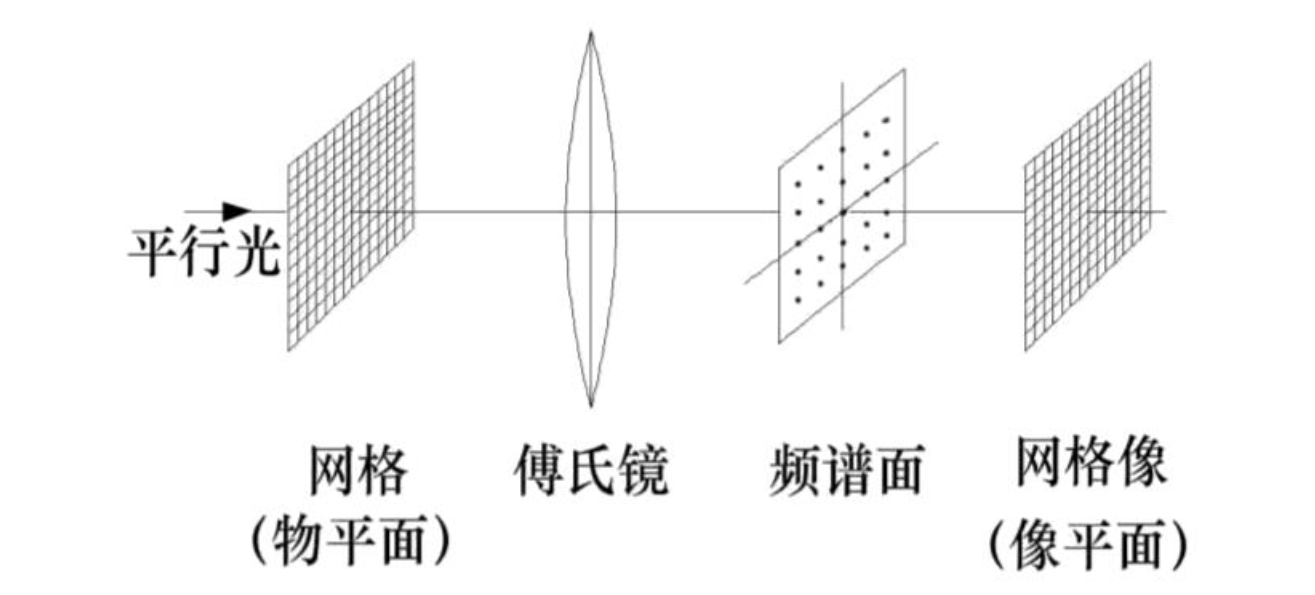二、傅里叶变换的应用

通过傅里叶变换将图像从事于转换到频域，然后进行相应的处理，例如滤波和增强等，然后再通过傅里叶反变换将图像从频域转换到时域，就可以实现图像的处理。 在这里 介绍一种滤波器：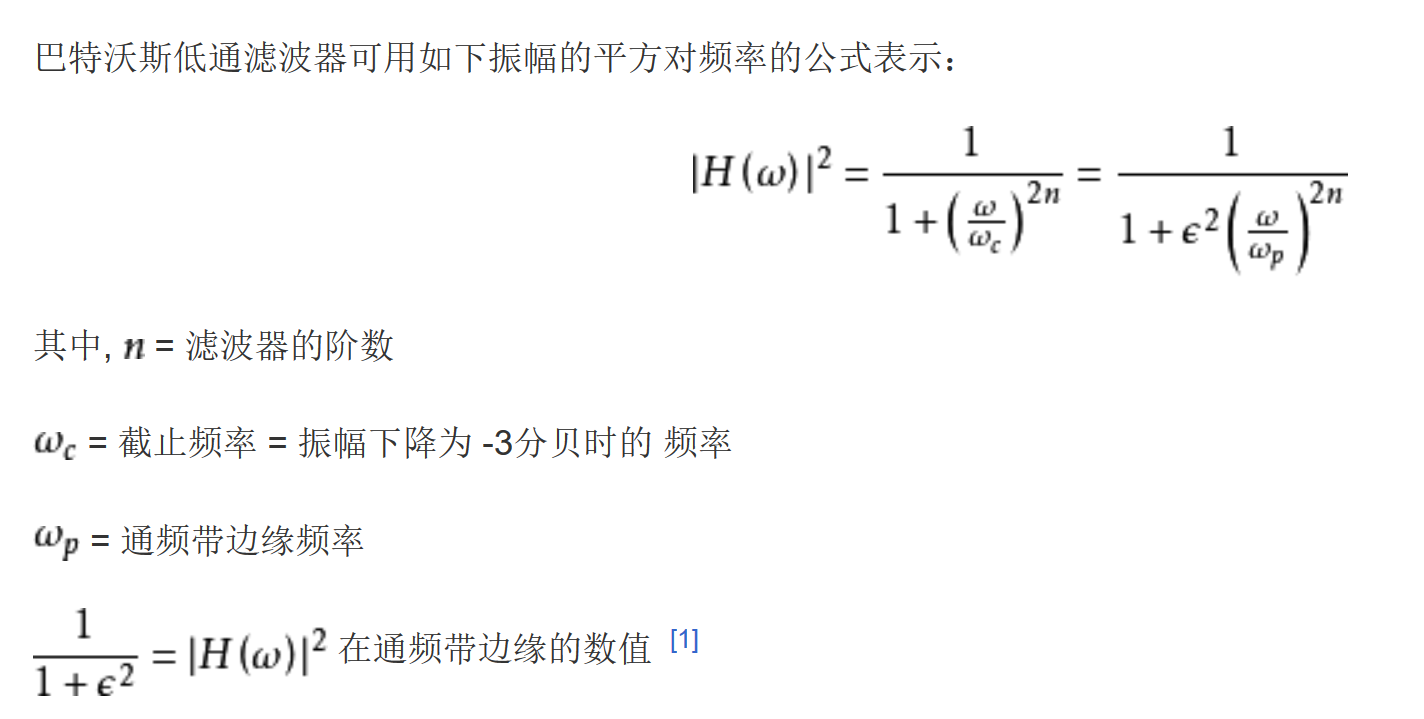用低通滤波处理图像：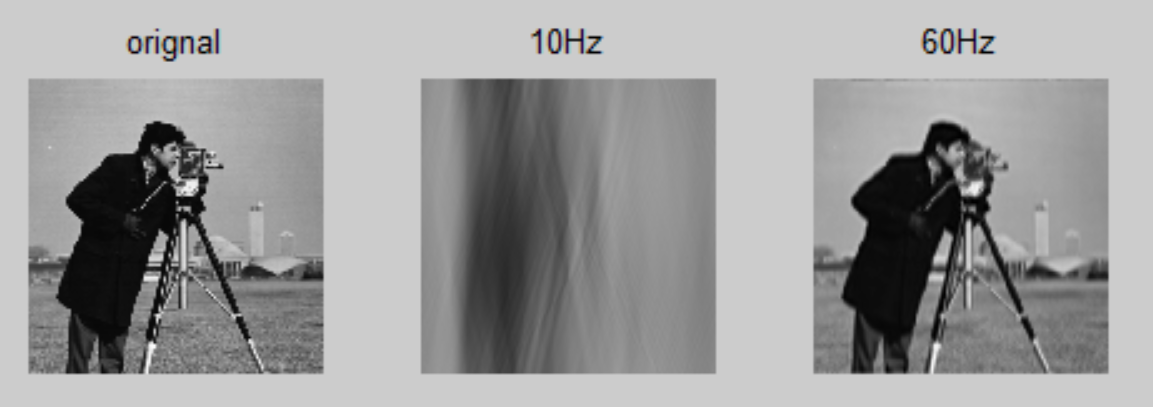可以看到滤波器的截止频率越低，图像越模糊；截止频率越高，滤波的效果越好。

程序：

i=imread('F:\matlab\MATLAB上机操作\图形\data\cameraman.tif');
i=im2double(i);
j=fftshift(fft2(i));
[x,y]=meshgrid(-128:127,-128:127);%产生离散数据
z=sqrt(x.^2+y.^2);
d1=10;d2=60;%滤波器截止频率
n=6;%滤波器的阶数
h1=1./(1+(z/d1).^(2*n));
h2=1./(1+(z/d2).^(2*n));
k1=j.*h1;
k2=j.*h2;
l1=ifft2(ifftshift(k1));
l2=ifft2(ifftshift(k2));
figure
subplot(131);imshow(i);title('orignal')
subplot(132);imshow(real(l1));title('10Hz')
subplot(133);imshow(real(l2));title('60Hz')


展开全文• 最新课件 %傅里叶变换 clc;clear all;close all; tic Fs=128%采样频率频谱图的最大频率 T=1/Fs%采样时间原始信号的时间间隔 L=256%原始信号的长度即原始离散信号的点数 t=(0:L-1*T%原始信号的时间取值范围 x=7*cos(2...
• frft(离散傅里叶分数变换)的matlab实现代码。利用其对单分量、多分量的LFM信号进行了检测和估计，结果验证了frft的正确性。
• 傅里叶变换matlab FFT 函数解析 傅里叶变换网上有很多资料了，本博客主要讲实现《信号系统》P181页，不同周期的方波 的傅里叶级数： 与sinc形成包络： 其间隔ω0，如下图： 本博客用matlab 实现一下。 下面...
傅里叶变换 和 matlab FFT 函数解析
傅里叶变换网上有很多资料了，本博客主要讲实现《信号系统》P181页，不同周期的方波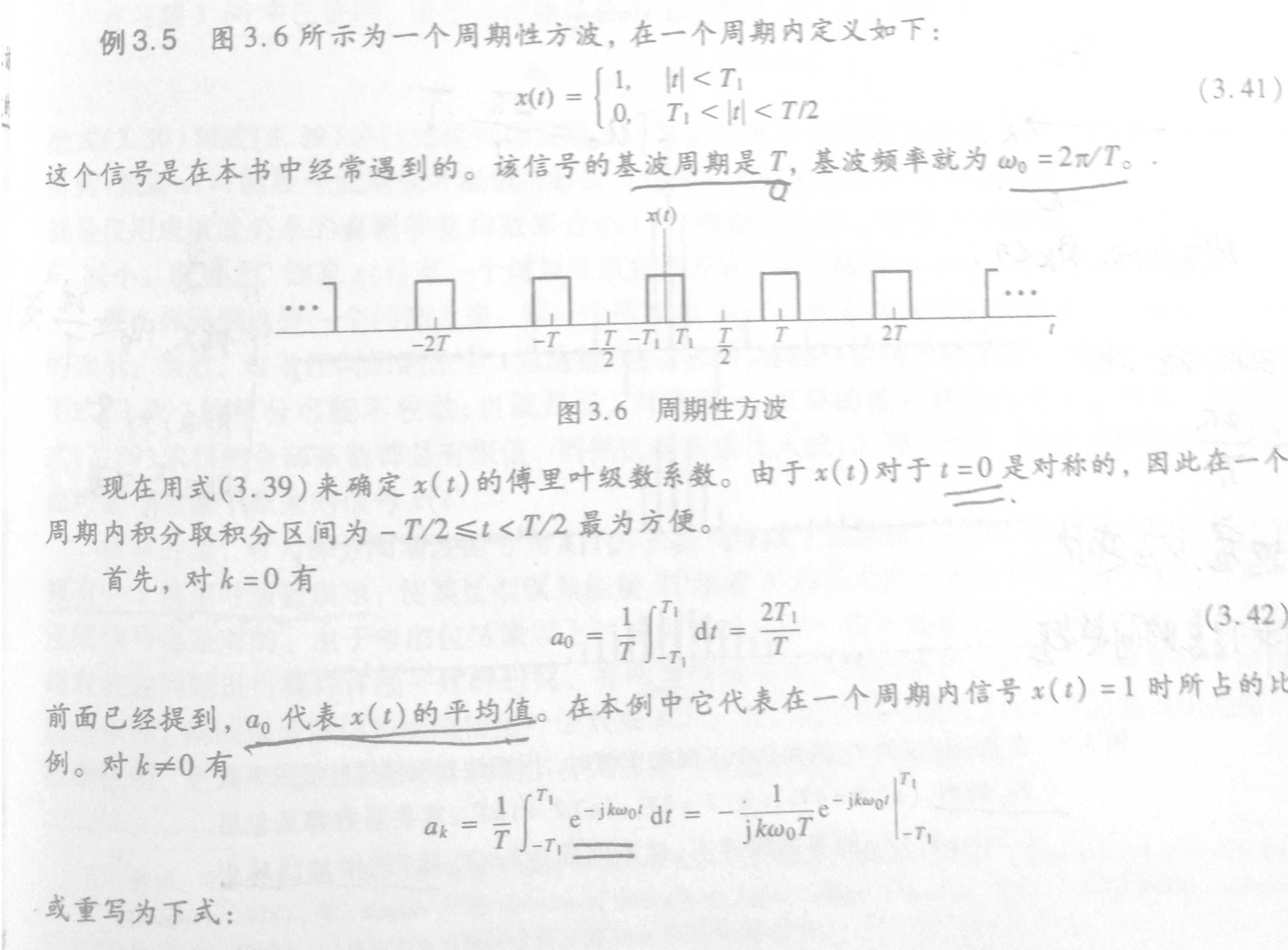的傅里叶级数：与sinc形成包络：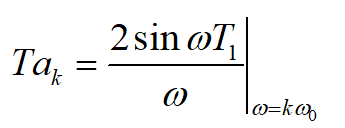其间隔ω0，如下图：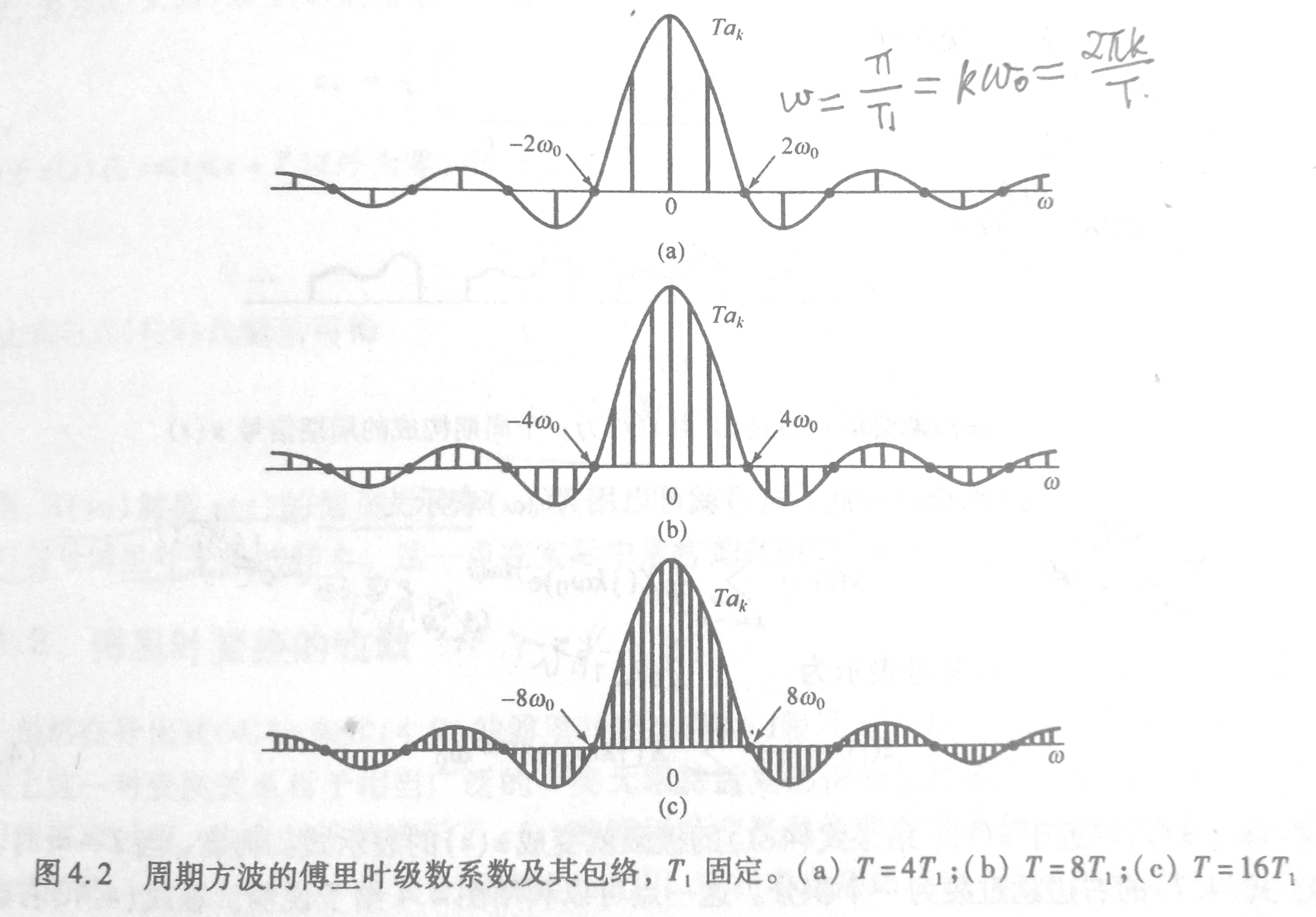本博客用matlab 实现一下。
下面解析matlab fft
matlab fft 函数用于离散快速傅里叶变换，输出一个与输入等长n的序列。
它按以下方式计算：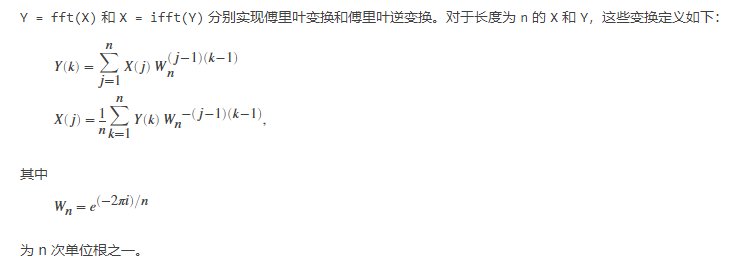有此公式也可以自行实现fft，例如实部：
clear
t0 = 0.1;% 采样间隙
tr = 32;
t = -tr:t0:tr;
n = length(t);
y = 3*cos(2*t);
mY = zeros(size(t));
w = linspace(-1/t0*pi,1/t0*pi,n);
for k = 1:n
for j = 1:n
mY(k) = mY(k) + y(j)*cos(-2*pi*(j-1)*(k-1)/n);
end
end
Y = fft(y);
plot(real(Y)-mY)

对比连续的傅里叶级数展开公式：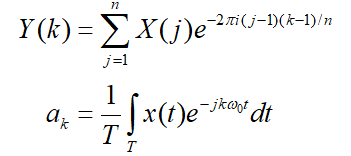设n是全部样本数，t0是时间间隔，则有以下转换方式：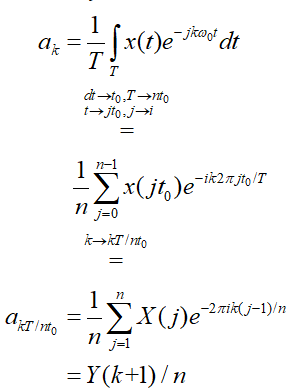那么傅里叶系数ak对应fft()函数结果有如下关系：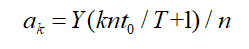这说明ak在fft()结果中间隔nt0/T，可以离散fft用来离散化连续傅里叶级数。
代码
基础设置
clear
t0 = 0.01;% 采样间隙
tr = 3200;
t = -tr:t0:tr;%样本时长，注意tr必须为T的整数倍，保证周期完整！
T1 = 0.1;%单周期方波时长
T = T1*16;%方波周期，改变T为4T1,8T1,16T1对应上面三个图
n = length(t); %离散化的点数，也对应fft结果的点数
y = zeros(size(t)); %信号
w = linspace(-1/t0*pi,1/t0*pi,n);% 频域范围对应2pi/t0
% ω 间隔2pi/(t0n),对应ak间隔2pi/(t0n)*nt0/T= ω0 与书上结论一致

产生占空比为2T1/T的方波：
for i = 1:n
if abs((rem(t(i),T))) < T1 || abs((rem(t(i),T)))>=T-T1
y(i) = 1;
end
end
figure()
plot(t,y);

调整T，画包络图
figure()
plot(w,2*sin(w*T1)./w) %sinc
hold on
Y = fft(y)/n;
k = fftshift(Y); %fftshift 将Y(0)移动到中心，Y(n/2:end)移动到负数频域
plot(w,T*real(k)) %频谱（包含ak，间隔nt0/T）

实验结果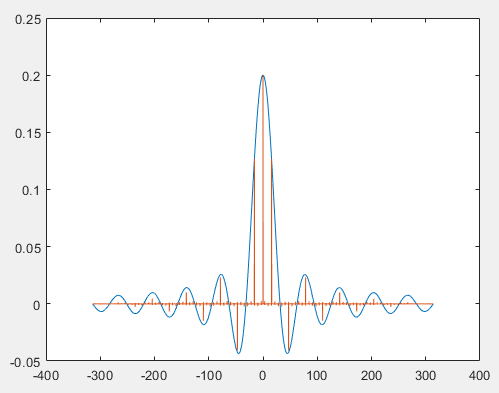T = 4T1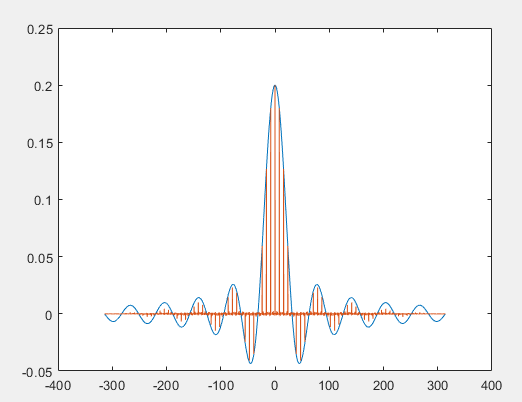T = 8T1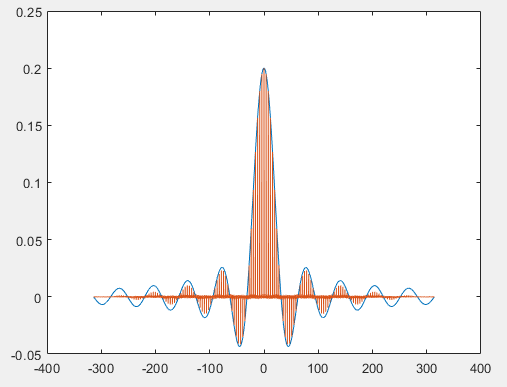T = 16T1
参考：

<<信号与系统>>
https://ww2.mathworks.cn/help/matlab/ref/fft.html


展开全文信号处理
• Fs = 1000; % Sampling frequency采样频率 T = 1/Fs; % Sample time采样周期 L = 1000; % Length of signal信号长度(点的个数) t = (0:L-1*T; % Time vector时间向量(序列(用来画图) % Sum of a 50 Hz sinusoid and ...
• 压缩包中包含原图和水印图片，可以使用，只是不知道是不是参数调整有问题，效果不是太好...

# 傅里叶变换matlabmatlab 订阅